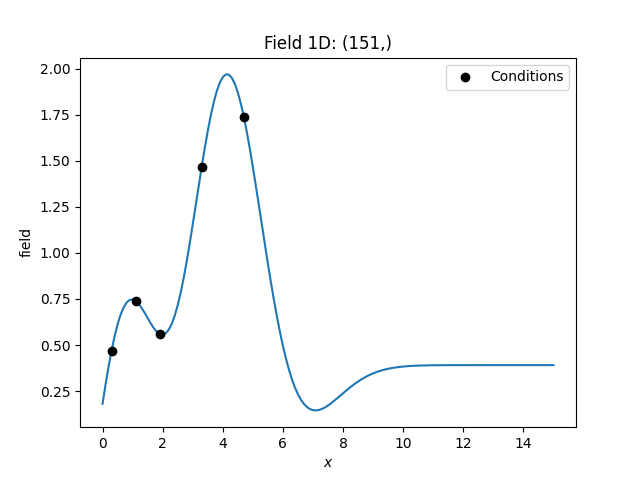# Ordinary Kriging¶

Ordinary kriging will estimate an appropriate mean of the field, based on the given observations/conditions and the covariance model used.

The resulting system of equations foris given by:Therebyis the semi-variogram of the given observations andis a Lagrange multiplier to minimize the kriging error and estimate the mean.

## Example¶

Here we use ordinary kriging in 1D (for plotting reasons) with 5 given observations/conditions. The estimated mean can be accessed by krig.mean.

import numpy as np
from gstools import Gaussian, krige

# condtions
cond_pos = [0.3, 1.9, 1.1, 3.3, 4.7]
cond_val = [0.47, 0.56, 0.74, 1.47, 1.74]
# resulting grid
gridx = np.linspace(0.0, 15.0, 151)
# spatial random field class
model = Gaussian(dim=1, var=0.5, len_scale=2)

krig = krige.Ordinary(model, cond_pos=cond_pos, cond_val=cond_val)
krig(gridx)

ax = krig.plot()
ax.scatter(cond_pos, cond_val, color="k", zorder=10, label="Conditions")
ax.legend()Total running time of the script: ( 0 minutes 0.118 seconds)

Gallery generated by Sphinx-Gallery